Reflection Of Light - Physics
06-01-2020    82 times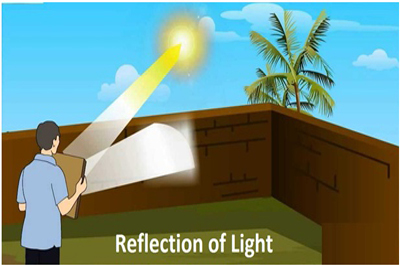### What is reflection of the light?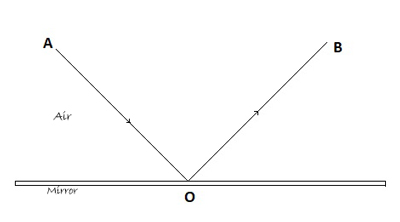If a light ray or beam is striking on a different medium, after traveling on another homogeneous medium, and then some portion of the light ray bouncing back to the first medium by changing the direction. This phenomenon is known as a reflection of the light.

In the figure, a light ray starts from point A in the air, and drop on the point O in the second media (mirror). And it returns back or reflects in the air media from the line OB. Here second media or mirror is known as a reflector.

### Regular Reflection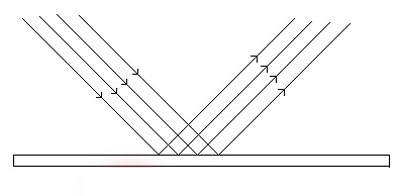If a parallel beam of light traveling through a medium, striking on a smooth surface, then it bounces back from it as a parallel beam of light in some other direction. This phenomenon is known as a regular reflection of the light.

### Irregular Reflection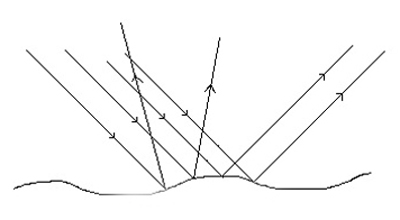If a parallel beam of light traveling through a medium, striking on a rough surface, then it bounces back from it as a non-parallel beam of light in a different direction. This phenomenon is known as an irregular reflection of the light.

### Incident Ray

The ray of light falls the mirror’s reflecting surface is called incident ray. Here AO is the incident ray.

### Reflected ray

The ray of light which sends back by the mirror is called Reflected ray. Here OB is the reflected ray.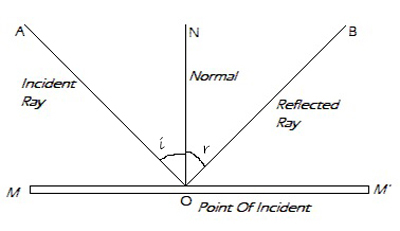### Point of incident

The point ( O ) at which the incident ray strikes the reflecting surface of the mirror is called the point of incident.

### Angle of incidence

The angle which the incident ray makes with the perpendicular line(ON) of the surface (called Normal) at the point of the incident is called the angle of incidence. Here ˂AON (i) is the angle of incidence.

### Angle of reflection

The angle which the reflected ray makes with the perpendicular line(ON) of the surface at the point of the incident is called the angle of reflection. Here ˂BON (r) is the angle of incidence.

### Law of Reflection

1. The angle of incidence and angle of reflection is equal
2. The incident ray, normal and reflected ray all lie in the same plane.